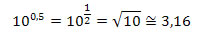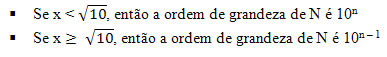Physicists Biographies

# Order of magnitude

When working with physical quantities, we often don’t need to worry about exact values. We can only approximate a result or measure. A feature that facilitates very long calculations in an evaluation is the use of orders of magnitude.

By definition, an order of magnitude of a number is the nearest power of ten to that number. Thus, to obtain the order of magnitude of any number N, we must first write it in scientific notation, that is, in the format:

N = x .10 n

where 1 ≤ x ≤10 and  n is an integerPower scale graph

Notice thatis approximately the midpoint of the interval [10 0 , 10 1 ] on a logarithmic scale. From this comparison,